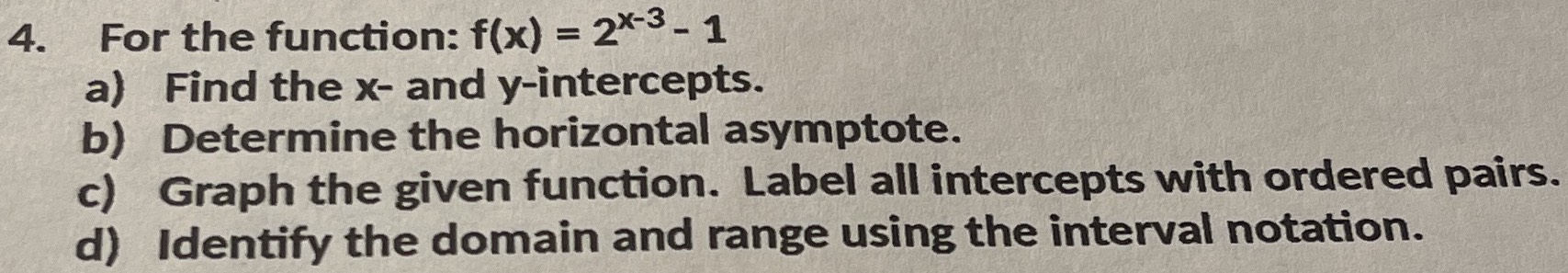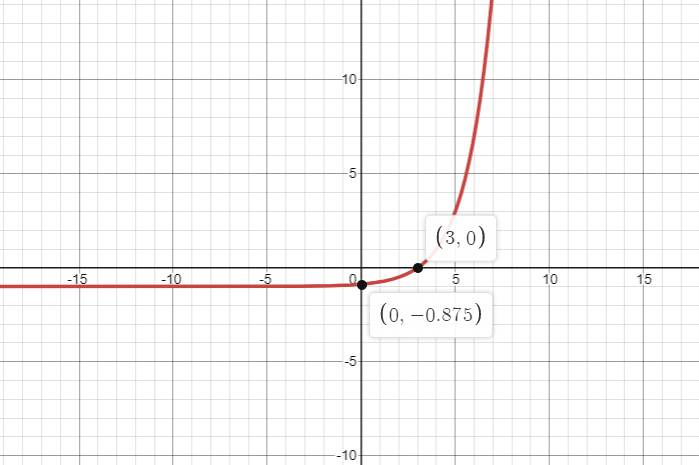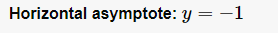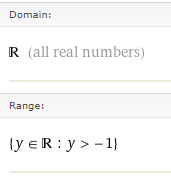### ¿Todavía tienes preguntas de matemáticas?

Pregunte a nuestros tutores expertos
Algebra
Pregunta4. For the function: $$f ( x ) = 2 ^ { x - 3 } - 1$$

a) Find the $$x$$ - and $$y$$ -intercepts. b) Determine the horizontal asymptote. c) Graph the given function. Label all intercepts with ordered pairs. d) Identify the domain and range using the interval notation.